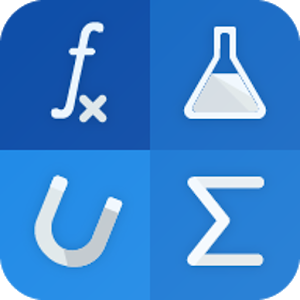Italiano

Ogni giorno offriamo licenze per app e giochi Android che altrimenti dovresti acquistare.1.49

# Android Giveaway of the Day - Formula Solver

Simple application for physical and chemical calculations.
User Rating: 0 (0%) 0 (0%)

Simple application for physical and chemical calculations. This app help you in physics, math and chemistry, if you need to know, what formula you must use in calculation, help you to control your calculation and calculating in formula.

Categories of physics:
* Electricity and magnetism
* Hydromechanics
* Mechanics of vibration, waves and acoustics
* Mechanics
* Molecular physics and ternodynamics
* Optics
* Quantum physics
* Special theory of relativity

Mathematical formulas

* For most calculations, it is possible to show the calculation procedure
* Search in categories
* Unit converter

Preparing:
* Show calculation procedure for all formulas (Premium)
* Add a formula to the test section
* Math formulas

The icons in the application come from www.materialdesignicons.com

The application icon is from Martin Šenovský - https://www.facebook.com/SenovskyMartin/

David Šimák

Education

3.0.0

3.1M

Everyone

4.0.3 and up

## Commenti su Formula Solver

Thank you for voting!